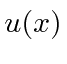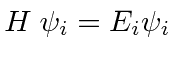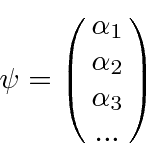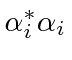## Eigenvalue Equations

The time independent Schrödinger Equation is an example of an Eigenvalue equation.The Hamiltonian operates onthe eigenfunction, giving a constantthe eigenvalue, times the same function. (Eigen just means the same in German.)

Usually, for bound states, there are many eigenfunction solutions (denoted here by the index).For states representing one particle (particularly bound states) we must require that the solutions be normalizable. Solutions that are not normalizable must be discarded. A normalizable wave function must go to zero at infinity.In fact, all the derivatives ofmust go to zero at infinity in order for the wave function to stay at zero.

We will prove later that the eigenfunctions are orthogonal to each other.We will assume that the eigenfunctions form a complete set so that any function can be written as a linear combination of them.(This can be proven for many of the eigenfunctions we will use.)

Since the eigenfunctions are orthogonal, we can easily compute the coefficients in the expansion of an arbitrary wave function.We will later think of the eigenfunctions as unit vectors in a vector space. The arbitrary wave functionis then a vector in that space.It is instructive to compute the expectation value of the Hamiltonianusing the expansion ofand the orthonormality of the eigenfunctions.We can see that the coefficients of the eigenstates represent probability amplitudes to be in those states, since the absolute squares of the coefficientsobviously give the probability.

Jim Branson 2013-04-22